Matlab实现简单的语音信号处理

一、实验目的

1.学习并掌握数字信号处理的基本方法。
2.学会使用MATLAB对语音信号进行处理。
3.学习并掌握MATLAB设计滤波器的方法。

二、实验内容

1.音频的录制与导入

2.语音信号的时域分析和频域分析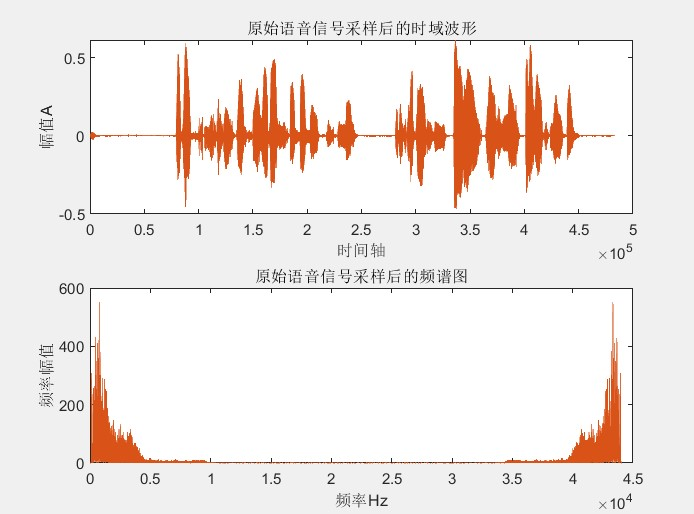3.设计滤波器对语音信号进行处理
（1）低通滤波器的设计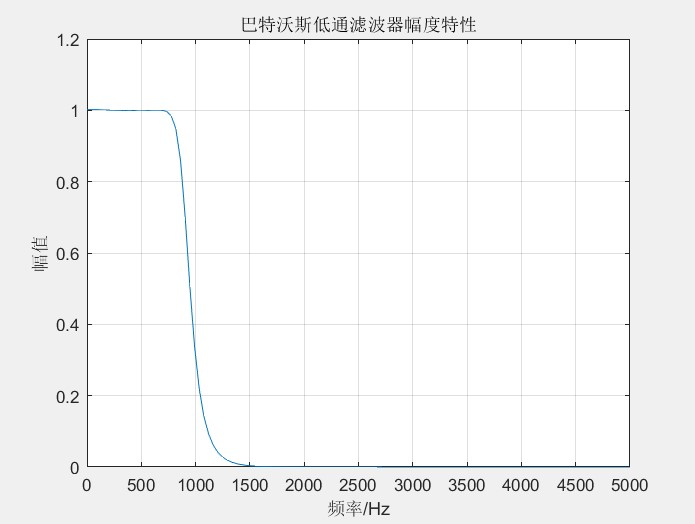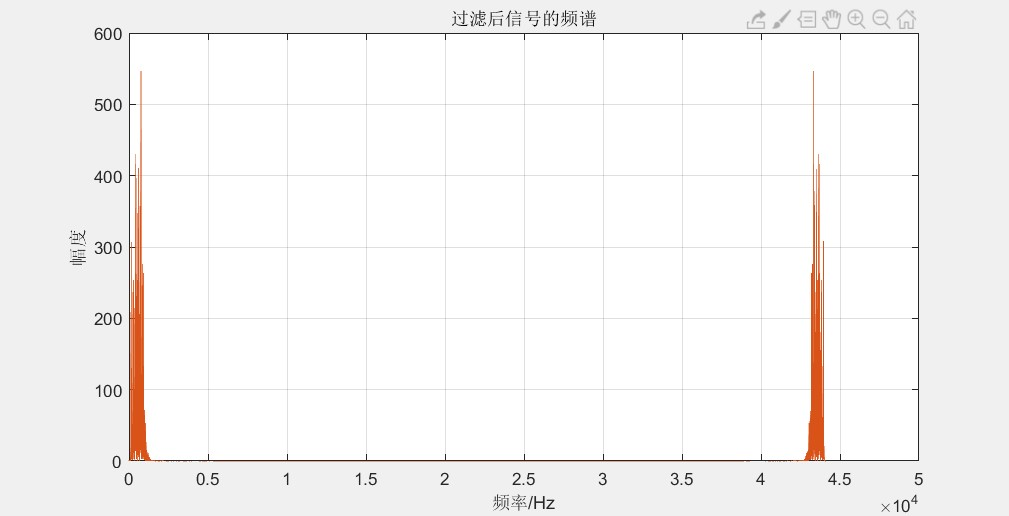（2）高通滤波器的设计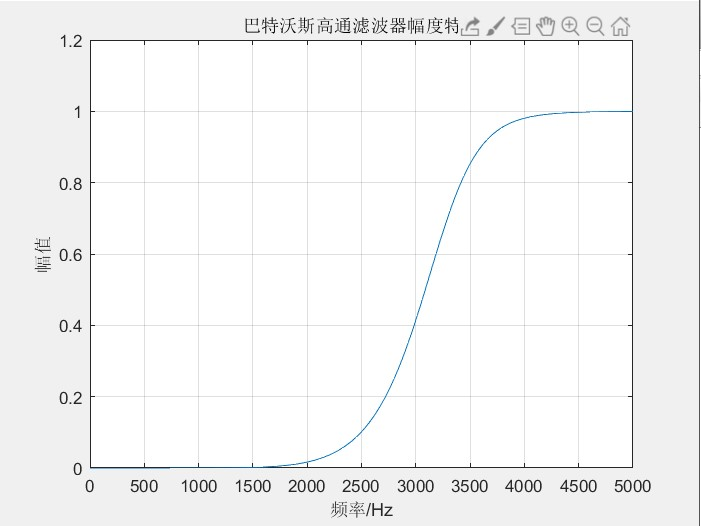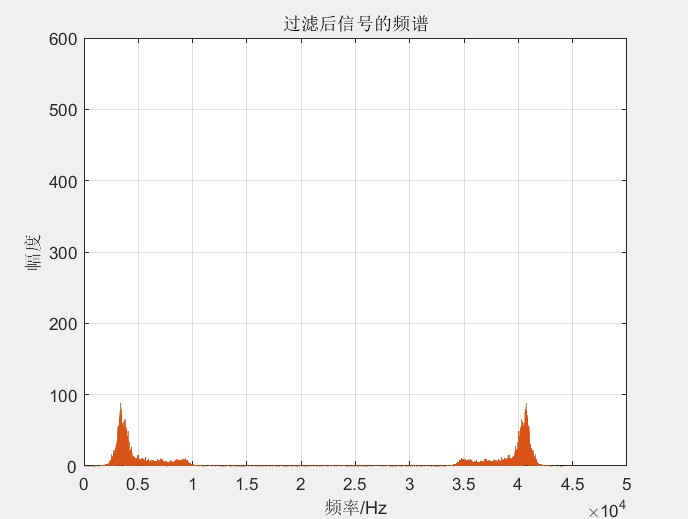（3）陷波器的设计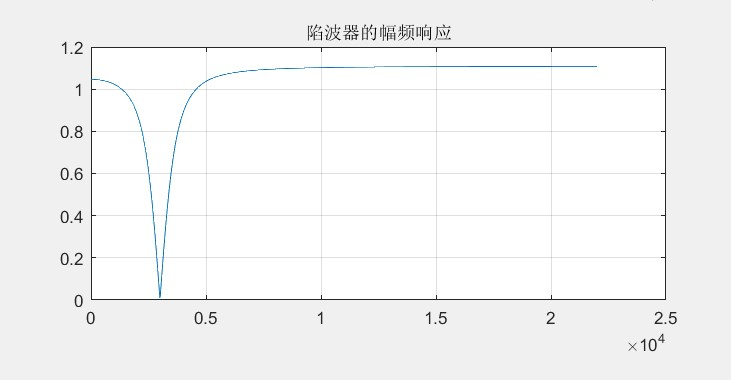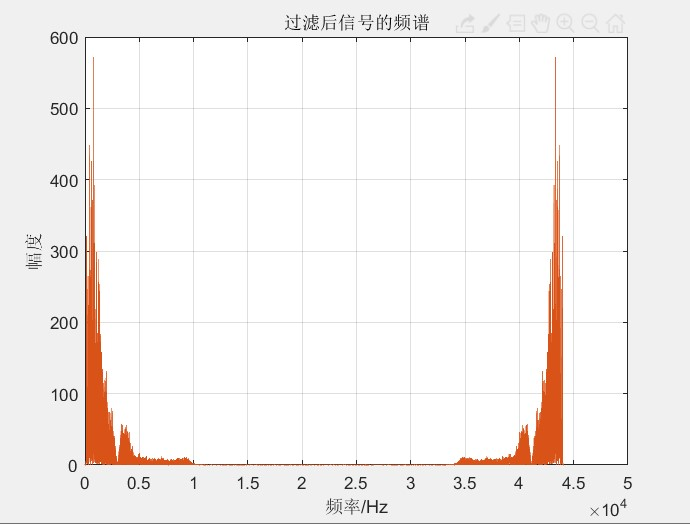（4）对语音信号加噪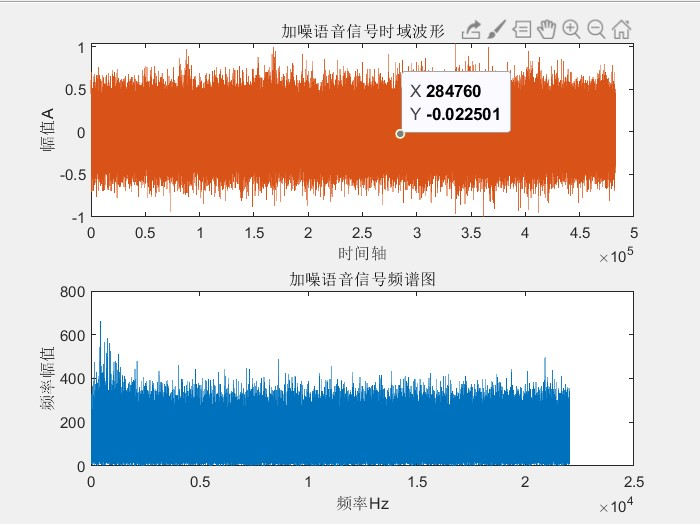三、实验代码

n=length(x);%获取语音信号的长度
x_p=fft(x,n);%利用FFT算法对语音信号进行离散傅里叶变换
f=fs*(0:n-1)/n;%频率的归一化
figure(1);%绘制时域和频域波形
subplot(2,1,1);
plot(x);
title(‘原始语音信号采样后的时域波形’);
xlabel(‘时间轴’)
ylabel(‘幅值A’)
subplot(2,1,2);
plot(f,abs(x_p));
title(‘原始语音信号采样后的频谱图’);
xlabel(‘频率Hz’);
ylabel(‘频率幅值’);
%噪声
% noise=0.2randn(1,n);
% x_z=x+noise’;
% sound(x_z,fs);
% n=length(x);
% x_zp=fft(x_z,n);
% f=fs
(0:n/2-1)/n;
% figure(5);
% subplot(2,1,1);
% plot(x_z);
% title(‘加噪语音信号时域波形’);
% xlabel(‘时间轴’)
% ylabel(‘幅值A’)
% subplot(2,1,2);
% plot(f,abs(x_zp(1:n/2)));
% title(‘加噪语音信号频谱图’);
% xlabel(‘频率Hz’);
% ylabel(‘频率幅值’);
% %低通滤波器
fp=800;fs=1300;rs=35;rp=0.5;Fs=44100;
wp=2Fstan(2pifp/(2Fs));%预畸
ws=2
Fstan(2pifs/(2Fs));
[n,wn]=buttord(wp,ws,rp,rs,’s’);
[b,a]=butter(n,wn,’s’);
[num,den]=bilinear(b,a,Fs);%双线性变换法 模拟滤波器转数字滤波器
[h,w]=freqz(num,den,512,Fs);
% 512为点数 Fs为频率范围
figure(2)
plot(w,abs(h));
xlabel(‘频率/Hz’);ylabel(‘幅值’);
title(‘巴特沃斯低通滤波器幅度特性’);
axis([0,5000,0,1.2]);
grid on;%网格线
%高通滤波器
% fp=3800;fs=3300;rs=3;rp=0.5;Fs=44100;
% wp=2Fstan(2pifp/(2Fs));%预畸
% ws=2
Fstan(2pifs/(2Fs));
% [n,wn]=buttord(wp,ws,rp,rs,’s’);
% [b,a]=butter(n,wn,’high’,’s’);
% [num,den]=bilinear(b,a,Fs);%双线性变换法 模拟滤波器转数字滤波器
% [h,w]=freqz(num,den,512,Fs);
% % 512为点数 Fs为频率范围
% figure(2)
% plot(w,abs(h));
% xlabel(‘频率/Hz’);ylabel(‘幅值’);
% title(‘巴特沃斯高通滤波器幅度特性’);
% axis([0,5000,0,1.2]);
% grid on;%网格线
%陷波器
% f0=3000;Fs=44100;r=0.9;
% w0=2pif0/Fs;
% num=[1 -2cos(w0) 1];
% den=[1 -2
rcos(w0) rr];
% N=1024;
% [H,w]=freqz(num,den,N);
% plot(w/pi/2Fs,abs(H));
% grid on;
% title(‘陷波器的幅频响应’);
% %x1=s1(:,1);%选取一个声道的数据
% x1=s1;
% %sound(x1,Fs);
% N1=length(x1);
% Y1=fft(x1,N1);
% f1=Fs
(0:N1-1)/N1;
% %t1=(0:N1-1)/Fs;
% figure(3)
% plot(f1,abs(Y1))
% xlabel(‘频率/Hz’);ylabel(‘幅度’);
% title(‘原始信号频谱’);
% grid on;axis([0 50000 0 600])
y=filter(num,den,x);
%sound(y,Fs);
N2=length(y);
Y2=fft(y,N2);
f2=Fs*(0:N2-1)/N2;
%t2=(0:N2-1)/Fs;
figure(4)
plot(f2,abs(Y2))
xlabel(‘频率/Hz’);ylabel(‘幅度’);
title(‘过滤后信号的频谱’);
grid on;
axis([0 50000 0 600])

目录